﻿ Sunshine's Homepage - 2D Line intersection
2D Line Intersection Example

This page explains and demonstrates the calculation of the intersection of two-dimensional lines using Vector analysis and the parametric form of vectors. Also the perp dot product is used in the calculation.

Following buttions can be used to load predefined line positions for the different cases:

### Usage:

This example application demonstrates the described algorithm for line intersection. The four red points defining the two lines can be freely dragged using the left mouse button to change their positions. The calculated result is updated automatically.

### Description:There are two lines specified by four points:
The infinite line gA is defined by the points A0 and A1. The line segment between A0 and A1 is called segA and its vector is further noted as a.
The same applies accordingly to B0, B1, gB and segB and b.

This can be formally written as following, introducing also vector notation:

• gA(s) = A0 + s * (A1 - A0) = A0 + s * a
where a is A1 - A0. If s is in [0,1], it defines a point of the line segment segA. With s = 0 point A0 is defined and with s = 1 point A1.
• gB(t) = B0 + t * (B1 - B0) = B0 + t * b (where vector b denoted as b is B1 - B0).
If t is in [0,1], it defines a point of the line segment segB. With t = 0 point B0 is defined and with t = 1 point B1.

Both lines and line segments can have one of the following relations:

• Collinear and true parallel: This means that both lines are parallel, but do not coincide. They have no intersection at all.

• Collinear and coincident: Both infinite lines are the same, they lie on each other. If line segments are considered instead of infinite lines, then following sub cases exist:
• The line segments overlap, either partly on one of the two sides or one line segment is even completely part of the other line segment.
• The line segments do not overlap, so they lie on the same infinite line both have not a single point in common.

• Intersect / Non-parallel The lines are skew, so there is exactly one intersection both. If line segments are considered instead of infinite lines, then following sub cases exist:
• The intersection point lies inside both line segments.
• The intersection point lies inside one line segment, but not inside the other line segment.
• The intersection point lies outside both line segment, but the intersection point exists on the infinite line.

### Parallel lines

If both lines are parallel, then also a and b have the same or opposite direction and thus are linearly dependent.
To check this case the perp dot product can be used: In this case, the perpendicular vector a to a must be also perpendicular to b. In other words, the perp dot product of a and b is zero.

In short: Lines are parallel if perpDot(a, b) = 0.

#### True parallel or coincident?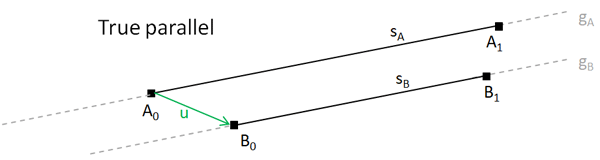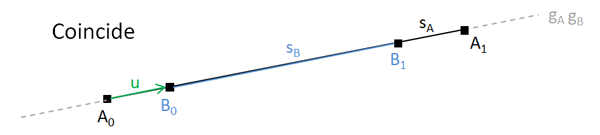If both lines are parallel, they could either be true parallel meaning they do never intersect or they are coincident meaning they lie on each other and are in fact the same line.

To check if both lines are the same, one point can be set into the equation of the other line to test if it lies also on the other line.
So e.g. we could set B0 into the line gA, that is B0 = A0 + s * a for some s.
Now let's define a vector u as u = B0 - A0. If both lines are coincident, A0 and B0 lie on the same line, meaning that u = s * a for some s.
In other words, u is linearly dependent to a, thus u must be also perpendicular to a, that is the perp dot product of u and a is zero.

In short: Lines are coincide if perpDot(a, u) = 0. Otherwise they are true parallel.

#### Coincident line segments with or without overlap

However, if line segments are considered (and not infinite lines), then coincidence can still means two cases: Either the line segments overlap or they do not overlap.To distinigush both cases, solve the line equation of onbe line for the end points of the other line segment and check if both parameters are in range [0, 1].
This can be done in following way:
Put B0 in gA, so solve B0 = A0 + s(B0) * a. This gives s(B0) = a / (B0 - A0).
If s(B0) is in range [0, 1], then B0 lies on the line segment between A0 and A1, else not.
Repeat the same steps for B1, resulting in s(B1) = a / (B1 - A0). If s(B1) is in range [0, 1], then B1 lies on the line segment between A0 and A1, else not.

In total, this means that both line segments partly overlap if either s(B0) is in range [0, 1] or s(B1) is in range [0, 1].
Both line segments totally overlap, so one line segment is completely contained by other line segments if s(B0) and s(B1) are in range [0, 1].
Both line segments are coincide but have no overlap at all, if neither s(B0) nor s(B1) are in range [0, 1].

### Non-parallel lines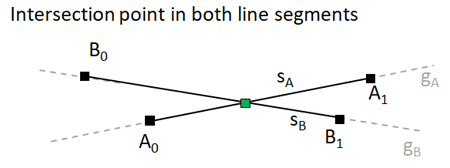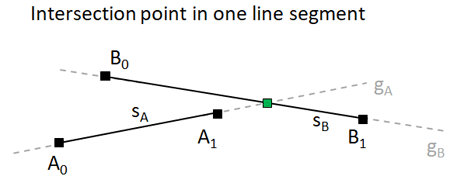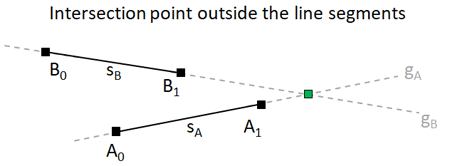If both lines are not parallel, then there exists exactly one intersection point.
To calculate the intersection point, we need to find the point at which both line equations are equal: gA(s) = gB(t). This means we need to calculate either s or t.
Let's have a closer look:

gA(s) = gB(t) ---> A0 + s * a = B0 + t * a

Lets's solve it for s. Therefore first isolate the s term:
s * a = (B0 - A0) + t * a
Now comes a trick: Multiply both sides with b. Because b * b is 0, this eliminates t:
s * b * a = b * (B0 - A0) + t * b * a
-> s * b * a = b * (B0 - A0)
-> s = ( b * (B0 - A0 ) / ( b * a )
Defining vector B0 - A0 as u, this is the same as s = perpDot(b, u) / perpDot(b, a)
The actual intersection point is then calculated as p = A0 + s * a.

Note that we have chosen s arbitrarily - we could have also chosen t to solve for:
A0 + s * a = B0 + t * a
-> (A0 - B0) + s * a = t * a.
Now multiply both sides with a:
-> a * (A0 - B0) + s * a * a = t * a * a
-> a * (A0 - B0) = t * a * a
-> t = (a * (A0 - B0)) / (a * a)
Defining vector A0 - B0 as u, this is the same as t = perpDot(a, u) / perpDot(a, b)
The actual intersection point is then calculated as p = B0 + t * b.

To distinguish the three cases if the intersection point is on both line segments, one line segment or not at all on any of the two lines segments (but on the infinite lines), again the calculated values for s and t need to be evaluated:
If s is in range [0, 1] AND t is in range [0, 1], then the intersection point lies inside both line segments.
If s is in range [0, 1] OR t is in range [0, 1], then the intersection point lies on one line segment. If s is in range [0, 1], then it's on line segment segA, else it's on segB.
If neither s is in range [0, 1] nor t is in range [0, 1], the intersection point does not lie on any of both line segments.

Hope you liked it!
Sunshine2k, December 2k19

### History

2019/12/17: Initial release.